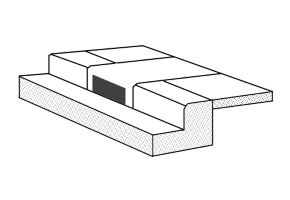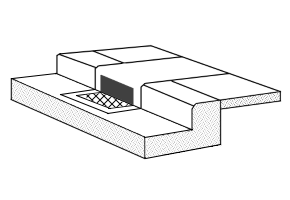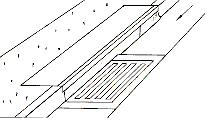Contents

An inlet on grade has a positive longitudinal gutter slope. Its interception capacity, Qi, is the flow intercepted by an inlet under a given set of conditions. The efficiency of an inlet, E, is the percent of total flow that the inlet will intercept for those conditions. The efficiency of an inlet changes with changes in cross slope, longitudinal slope, total gutter flow, and, to a lesser extent, pavement roughness. In mathematical form, efficiency, E, is defined by the following equation:

Where:
E = inlet efficiency
Q = total gutter flow, cfs (cms)
Qi = Intercepted flow, cfs (cms)

Flow that is not intercepted by an inlet is termed Bypass.

## Factors Affecting Interception Capacity

The interception capacity of inlet on grade increases with increasing flow rates, and inlet efficiency generally decreases with increasing flow rates. Factors affecting gutter flow also affect inlet interception capacity.

The depth of water next to the curb is the major factor in the interception capacity of both grate inlets and curb-opening inlets.

The interception capacity of a grate inlet depends on the amount of water flowing over the grate, the size and configuration of the grate and the velocity of flow in the gutter. The efficiency of a grate is dependent on the same factors and total flow in the gutter.

Interception capacity of a curb-opening inlet is largely dependent on flow depth at the curb and curb opening length. Flow depth at the curb and consequently, curb-opening inlet interception capacity and efficiency, is increased by the use of a local gutter depression at the curb opening or a continuously depressed gutter to increase the proportion of the total flow adjacent to the curb.

Top slab supports placed flush with the curb line can substantially reduce the interception capacity of curb openings. Tests have shown that such supports reduce the effectiveness of openings downstream of the support by as much as 50% and, if debris is caught at the support, interception by the downstream portion of the opening may be reduced to near zero. If intermediate top slab supports are used, they should be recessed several inches from the curb line and rounded in shape.

Slotted inlets function in essentially the same manner as curb opening inlets, i.e., as weirs with flow entering from the side. Interception capacity is dependent on flow depth and inlet length. Efficiency is dependent on flow depth, inlet length and total gutter flow.

The interception capacity of an equal length combination inlet consisting of a grate placed alongside a curb opening on a grade does not differ materially from that of a grate only. Interception capacity and efficiency are dependent on the same factors which affect grate capacity and efficiency.

A combination inlet consisting of a curb-opening inlet placed upstream of a grate inlet has a capacity equal to that of the curb-opening length upstream of the grate plus that of the grate, taking into account the reduced spread and depth of flow over the grate because of the interception by the curb opening. This inlet configuration has the added advantage of intercepting debris that might otherwise clog the grate and deflect water away from the inlet.

## Curb InletsInterception capacity of a curb-opening inlet is largely dependent on flow depth at the curb and curb opening length. Flow depth at the curb and consequently, curb-opening inlet interception capacity and efficiency, is increased by the use of a local gutter depression at the curb opening or a continuously depressed gutter to increase the proportion of the total flow adjacent to the curb.

The interception capacity for curb inlets is computed using the following equation. The equation also applies to slotted inlets.

Where:
LT = curb opening length for 100% capture in ft (m)
KT = 0.6 (0.817)
Q = gutter flow in cfs (cms)
SL = gutter slope, longitudinal in ft/ft (m/m)
n = Manning’s n-value
Se = equivalent cross slope

Where:
Sx = cross slope of pavement in ft/ft (m/m)
S’w = depression in ft (m) / gutter width in ft (m) or, for
non-depressed inlets, cross slope Sw – cross slope Sx
Eo = Ratio of flow in the gutter section to total gutter flow

If the specified inlet length is larger than LT, it will capture 100% of the flow and “Q captured will equal Q. If the specified inlet length is less than the computed LT, then Q captured is computed as follows:

Where:
QT = Q catchment + Q carryover
EF = 1 – (1 – L/LT)1.8 = Efficiency
Q bypassed equals QT – Q captured

## Grate Inlets

The interception capacity of a grate inlet on grade depends on the amount of water flowing over the grate, the size and configuration of the grate and the velocity of flow in the gutter just upstream. The efficiency of a grate is dependent on the same factors and total flow in the gutter.

The interception capacity of grate inlets on grade is computed using the following equations:

Where:
E = efficiency of the grate
Rf = ratio of intercepted frontal flow to total gutter flow
Eo = ratio of frontal flow to total gutter flow
Rs = side flow interception efficiency

The Rf term in the above equation is dependent on the specific grate properties illustrated in HEC-22 and most likely will not match every situation. In that light, Studio Express assumes Rf = 1. This assumes all frontal flow will be intercepted without any loss of flow due to splash-over effects.

Where:
Kc = 0.15 (0.0828)
V = velocity of flow in the gutter in ft/s (m/s)
L = grate length in ft (m)

The amount of intercepted flow for grates on grade = E x Q. Non-intercepted flows are bypassed.

## Combination InletsThe interception capacity of combination inlets on grade is essentially equal to that of the grate alone. Studio Express computes this capacity by neglecting the curb opening (per HEC-22) and using the methods described above for grate inlets.

Sweeper InletsWhen the curb opening length is longer than the grate length, the program assumes the open curb portion to be located upstream of the grate, often called a “sweeper” inlet. The sweeper inlet has an interception capacity equal to the sum of the curb opening upstream of the grate plus the grate capacity. The grate capacity in this case is reduced by the interception by the upstream cub opening.